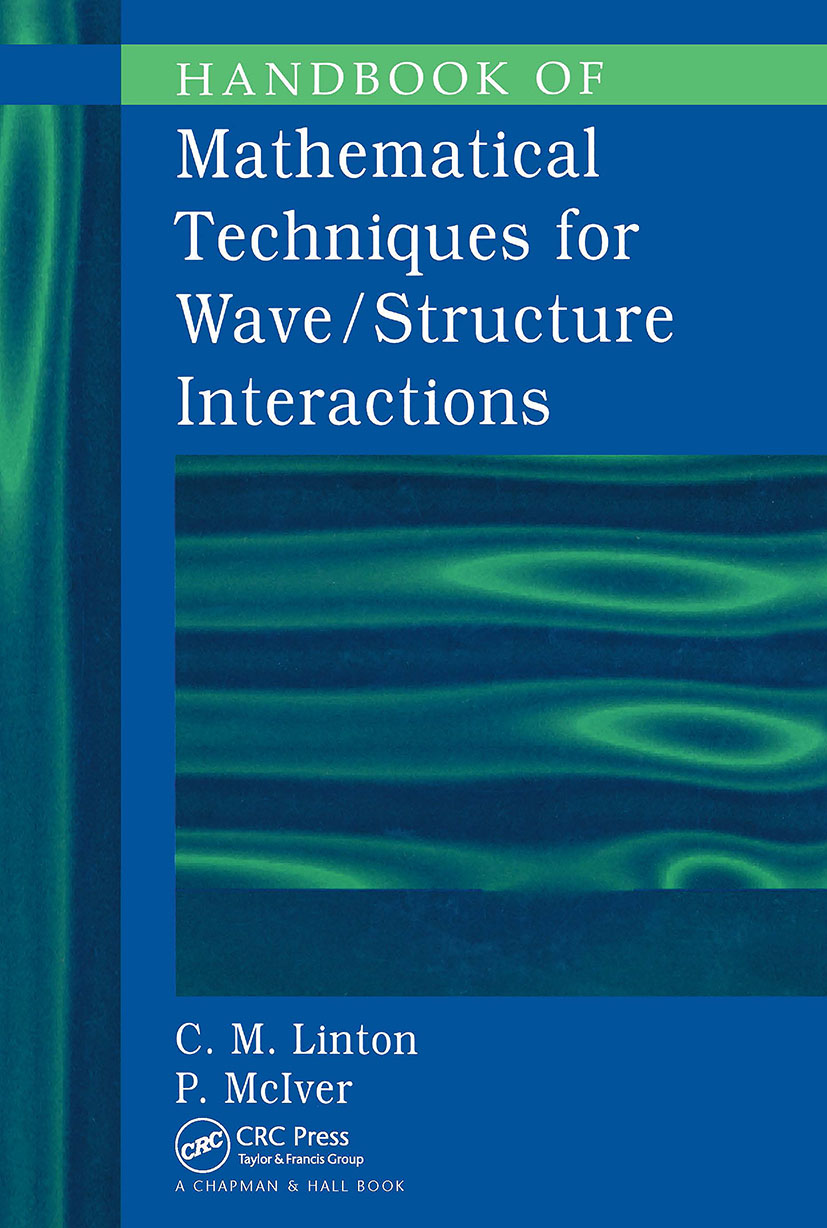# Handbook of Mathematical Techniques for Wave/Structure Interactions

## 1st Edition

Chapman and Hall/CRC

316 pages

##### Purchasing Options:\$ = USD
Hardback: 9781584881322
pub: 2001-02-26
\$175.00
x
eBook (VitalSource) : 9780429123603
pub: 2001-02-26
from \$28.98

FREE Standard Shipping!

### Description

Although a wide range of mathematical techniques can apply to solving problems involving the interaction of waves with structures, few texts discuss those techniques within that context-most often they are presented without reference to any applications. Handbook of Mathematical Techniques for Wave/Structure Interactions brings together some of the most important techniques useful to applied mathematicians and engineers.

Each chapter is dedicated to a particular technique, such as eigenfunction expansions, multipoles, integral equations, and Wiener-Hopf methods. Other chapters discuss approximation techniques and variational methods. The authors describe all of the techniques in terms of wave/structure interactions, with most illustrated by application to research problems. They provide detailed explanations of the important steps within the mathematical development, and, where possible, physical interpretations of mathematical results.

Handbook of Mathematical Techniques for Wave/Structure Interactions effectively bridges the gap between the heavy computational methods preferred by some engineers and the more mathematical approach favored by others. These techniques provide a powerful means of dealing with wave/structure interactions, are readily applied to relevant problems, and illuminate those problems in a way that neither a purely computational approach nor a straight theoretical treatment can.

INTRODUCTION

The Water-Wave Problem

The Linearised Equations

Interaction of a Wave with a Structure

Reciprocity Relations

Energy of the Fluid Motion

EIGENFUNCTION EXPANSIONS

Introduction

Construction of Vertical Eigenfunction

Two-Dimensional Problems

Three-Dimensional Problems

Matched Eigenfunction Expansions

MULTIPOLE EXPANSIONS

Introduction

Isolated Obstacles

Multiple Bodies

INTEGRAL EQUATIONS

Source Distribution

Green's Theorem

Thin Obstacles

Interior Problems

Free-Surface Problems

Numerical Evaluation of Green's functions

Diffraction by a Gap in a Breakwater

Diffraction by an Insular Breakwater

Embedding Formulae

Numerical Solutions

THE WIENER-HOPF AND RELATED TECHNIQUES

The Weiner-Hopf Technique

Residue Calculus Theory

ARRAYS

The Wide-Spacing Approximation

SMALL OBJECTS

Introduction

Breakwater with a Gap

Vertical Cylinder

Heaving Cylinder

Eigenvalue Problems

VARIATIONAL METHODS

Scattering and Radiation Problems

Eigenvalue Problems

APPENDICES

Bessel Functions

Multipoles

Principle Value and Finite Part Integrals

### Subject Categories

##### BISAC Subject Codes/Headings:
MAT003000
MATHEMATICS / Applied
TEC009020
TECHNOLOGY & ENGINEERING / Civil / General
TEC009070
TECHNOLOGY & ENGINEERING / Mechanical